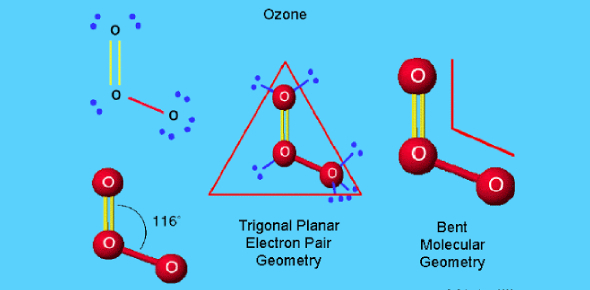# Chemistry Quiz: Molecular Geometry

11 Questions | Total Attempts: 32Settings.

• 1.
Which of the following play an important role in the reactivity of molecule?
• A.

The type of molecule

• B.

The number of atom in the molecule

• C.

The speed of the reaction

• D.

The shape of the molecule

• 2.
One of these molecules have 3 lone pairs bonded?
• A.

NH3

• B.

NaCl

• C.

H2O

• D.

BaCl2

• 3.
Every molecules have 3 atoms have a same reactivity?
• A.

Yes

• B.

No

• 4.
Of the following species , ___________ will have bond angles of 120?
• A.

PH3

• B.

ClF3

• C.

NCl3

• D.

BCl3

• E.

All of these following will have a bond angles of 120

• 5.
In H2O, what is the electron domain geometry? And what is the angle ?
• A.

Tetrahedral , 120

• B.

Linear , 180

• C.

Bent , 108.5

• D.

Tetrahedral , 109.5

• 6.
The electron domain geometry of ___________ is tetrahedral.
• A.

CBr4

• B.

PH3

• C.

CCl2Br2

• D.

XeF4

• E.

All of the above except XeF4

• 7.
The molecular geometry of the NO2*-1:
• A.

Trigonal planar

• B.

Linear

• C.

Tetrahedral

• D.

Bent

• 8.
Which of the following is polar:
• A.

NO2

• B.

CCl4

• C.

BF3

• D.

BCl3

• 9.
The _______ is the arrangement of only the atoms in a molecule?
• A.

Electron domain geometry

• B.

Molecular geometry

• C.

None of the above

• D.

All of the above

• E.

None of the above

• 10.
One of the following is polar:
• A.

BF3

• B.

CCl4

• C.

CH3Cl

• D.

None

• 11.
In O=C=O is the O=C polar?
• A.

Yes

• B.

No

Related TopicsBack to top